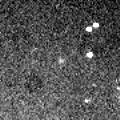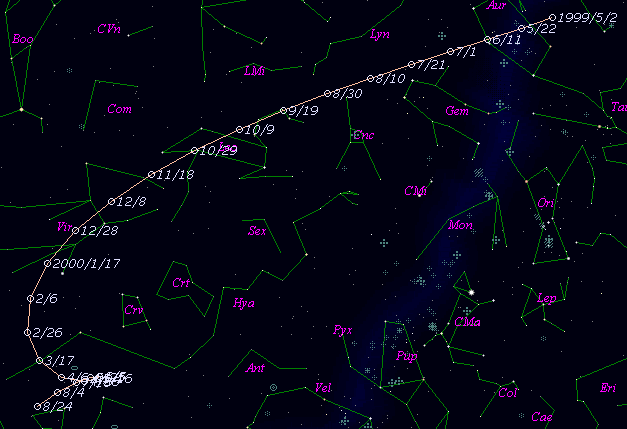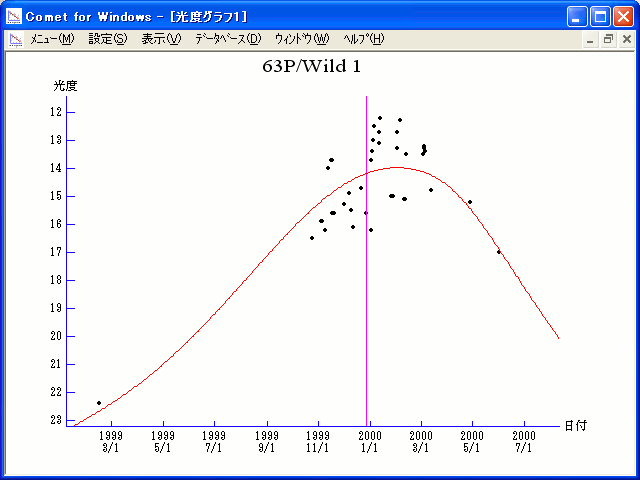# \$B%t%#%k%HBh#1WB@1(B

63P/Wild 1 (1999)###\$B###\$B50F;MWAG(B

```   The following improved orbital elements by Kenji Muraoka, are
from 55 observations  1960 to 1999, including 9 Planets, Moon
and 5 minor planets perturbations and non-gravitational effect
of style II.    The mean residual is +/- 0.72 arc seconds.

Epoch  =  1999 Dec.  8.0  TT       JDT = 2451520.5
T  =  1999 Dec. 27.08367       +/- 0.00087 (m.e.) TT
Peri. =  167.99112                +/- 0.00038
Node  =  358.52509                +/- 0.00010   (2000.0)
Incl. =   19.93399                +/- 0.00006
q  =    1.9608838              +/- 0.0000041 AU
e  =    0.6497111              +/- 0.0000008
a  =    5.5979045              +/- 0.0000030 AU
n  =    0.07441592             +/- 0.00000006
P  =   13.245                  +/- 0.0000106  years
A1  =   +0.702                  +/- 0.091
A2  =   -0.11267                +/- 0.00052
```

###\$B@1?^(B###\$B8wEYJQ2=(B

```        m1 = 4.0 + 5 log\$B&\$(B + 30.0 log r
```##### \$B50F;MWAG\$OB<2,7r<#;a\$N7W;;\$K\$h\$k\$b\$N\$G\$9!#(B \$B@1?^\$O(B StellaNavigator Ver.2.0 for Windows (\$B%"%9%H%m%"!<%D(B \$BJTCx(B / \$B%"%9%-!<=PHG6I4)(B) \$B\$G:n@.\$7\$?\$b\$N\$G\$9!#(B \$B8wEY%0%i%U\$O(BComet for Windows\$B\$G:n@.\$7\$?\$b\$N\$G\$9!#(B